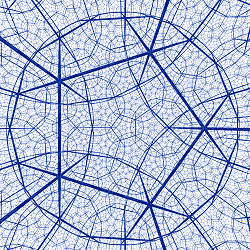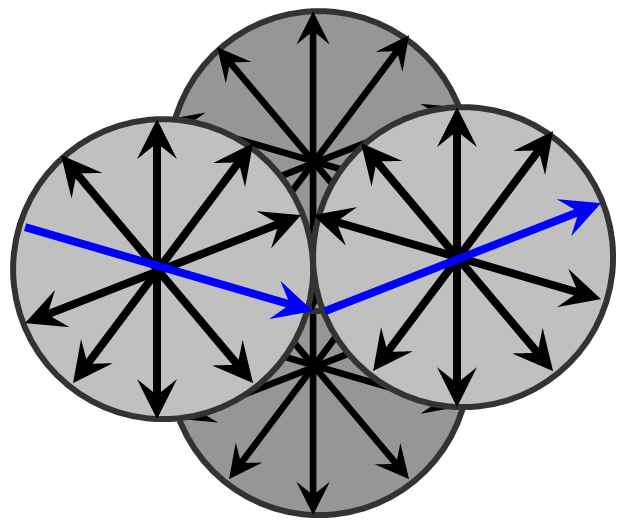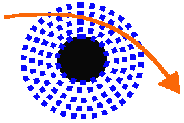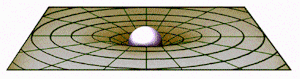Properties of Energy & Matter by James Clifford Cranwell 2/17/98 | Abstract| Dimensionality| Flux| Gravity| Atomic| Relativity| Big-Bang| Miscellaneous|

Change Font Size: A A A

 by James Clifford Cranwell 4/01/98 Part III ... GravityAs Star light travels through the Universe, what actually happens is a specific frequency or vibration (a photon) travels along one or more of the flux particle axes strings. The flux particles are arranged in a Field or matrix.
The matrix is held in place by the gravitational effect (the attraction of particles to each other) and curved around any mass in proximity. Light energy or electro-magnetic expansive force adds to the rigidity of the field matrix, but no matter how great the strength of any individual segment of a lattice structure, it is easily folded up or warped by any other force, in this case Gravity.

The DodecahedronThe Dodecahedron (DDH) is the pattern or axial arrangement used by the unit Flux, it is stackable hyperbolically and absolutely the most efficient way to pack space with the least amount of material (smallest angle of symmetrical invariance, any more than ten axes per particle and they won't connect).
The only problem is the vertices are tri-linear (where any axis terminates it will intersect with three others). If a frequency traveling along an axis passes directly through the center, then out any of the tri-corners, that same linear direction will not pass through any other of the twelve Dodecahedrons in proximity.It will be directly on the edge where any four Dodecahedrons meet. So the individual Photon which we now know is one vibration traveling along the Unit Flux strings can never take a straight path. The vertices where the Dodecahedrons meet have four paths.
If you place three base balls on the ground in a triangle then place another on top to make a pyramid shape, that will be the way the base balls and Dodecahedrons stack. The only problem here is where the base balls touch each other is not where the Unit Flux Strings are located. The end points the Flux Strings terminate on tri-corners. Thus sending the intrepid photon on a Zigzag, Corkscrew, and other intricate paths destined to scatter.
It is easy to angle out of position with nothing else around. It is the combined effect of many photons initially heading in one direction with momentum that keep each other in line and in proper direction except of course near the scattering outer fringes. This is the reason why one photon particle traveling in a zigzag can also be interpreted as wave function. Its path is forced into a wave type motion. A vibration sets off resonant vibrations in itself, perpendicular to the original path. Thus creating other fields and background radiation.

Graviton...38 Dimensions or 10-38 strengthDrawn here with spherical encasement for clarity, this is the way Flux pack space and are compressed in proximity to a large mass. To complete one wave of pull the gravitational attractor charge takes on its journey, one complete wave of the zigzag must be used to stay in any given direction (remember the Flux axes intersect in groups of four).
So for this package that's nine dimensional Flux dispersal times four unit Flux or 10-36 strength, if only two paths are used through the group that makes the dimension of the group 10-38 or Gravity. If we replace the G in Newton's equation F = Gm1m2 / r 2 with the exact value we get F = m1m2 / ((10 √26-1)c)r 2 then we can easily see the masses divided by the radius squared times the length in question equals the force of gravity.

No problem curving lightThe flux particle field is held in place and curved around any mass in proximity and pulled in and compressed thanks to the lattice type arrangement of the vertices. If the gravitational force is strong enough (an infamous (supposed) Black Hole made entirely of protons or neutrons... same thing et.al.) the Flux will flatten down to an electron like 2-D shape with the normal or volt spike included in the fold, giving the surface structure of the Black Hole the same functionality and appearance as a giant Electron in space.
Once again Newton's equation F = Gm1m2 / r 2 breaks down and becomes ineffective, the whole particle field shape and dimension has changed. The overall composition could be considered a gigantic atom in space. The Flux are completely compressed at the surface but make a smooth interdimensional transition back to a point in normal space. This means not only will light escape or discharge but it will be an immensely brighter light than is possible as the result of any Stars Hydrogen fusion reaction.
As you approach a Black Hole there will be a dramatic increase in gravity. The zigzag (lattice structure) of particle matrix axes increases, folding up like an accordion. The distance light has to travel increases thereby slowing it, compressing time and space along with it (from an unaffected observers reference frame). But it will take longer and longer to pass through the compressing field so, as the field compresses the overall effect is time will slow down and you will compress along with the field... you might not even notice (until it's too late), you will not stretch out.
(special note: Gravity is the attraction of particles pulling complete groups of atoms together. In a black hole there is a continuous proton / neutron pack and they have shedded their electrons, and although the protons / neutrons are held together with the strong force, i.e. one radius each curled up together, there is nothing to actually compress them together as a group. It would be something like a pearl necklace, any pearl might be strongly connected to its neighbor, but if you folded them up into a ball, there is nothing to collapse them together into a supposed singularity, they are connected but they are not pulling. )

Cosmological Constant: Fifth Force : Dark Energy : Quintessence : Æther : The Aether

The speed of waves of any system is given by the equation: Velocity "v" of propagation equals the square root of the quantity Tension "F" divided by the Mass "u" per unit length ... v = √( F / u ), squaring both sides we have: v 2 = F / u .
In our section of the universe, we know the velocity is the speed of light, so let's assume c2 = F / u . Even though the values for tension and mass are unknown, it's reasonable to assume the mass of space is vanishingly small, that makes the tension of the field tremendously greater than its mass (the ratio is the speed of light squared: 89,875,517,873,681,764 ).

When any flux particle is in free space it has vibration or light energy from billions and billions of stars traversing it in every possible direction. Although every particle is attracted to every other particle, without any large force or vibration on one specific axis, every axis will repel or expand away from every other axis, attempting to expand the whole field to its greatest volume. This process can be considered a universal expansive force, the Fifth Force or Dark Energy.

An easy to understand two dimensional model of the Flux Particle Field would be something like a highly stretched tennis net made of the finest spiders web, and in this model "matter" would be a balled up piece of the web stuck to the net (everything is infinitesimally small). This field is not stationary in space with other things rushing through it like MM mistakenly assumed. It is everywhere and surrounds matter. (note: it might be stationary way out in deep space where there are no planetary, solar and galactic, etc. Movements)

It works in the same type of manner as gravity (a group effort to push or pull in any particular direction) but opposite in force direction. Every particle attracts every other particle but that just creates the field. Nothing can actually happen until an irregularity is introduced into the system, for instance any particle becoming confined as proton mass. Then the field will curve around it and it will be drawn to and/or attract anything in its realm. Gravity and force five ratio varies at different places, for instance near a star there is gravity and a tremendous light energy or expansive force, but on Earth there is gravity and only residual sunlight (expansive force). Gravity ( the actual particle attractions) are the major factor in drawing in a mass and curving the field. The centripetal force along with expansion keep it at bay. Remember... no matter how strong an individual segment of lattice structure, the whole structure is easily curved or warped by any other force. (if something is packing itself around something curved, like the surface of any planet or star, it will... you guessed it, curve.)
Yes, all these things...
Cosmological Constant: Fifth Force : Dark Energy : Quintessence
... are what others have been unknowingly calling the the stretching property of Flux Particle Field.
The field itself (and any loose particles) which completely fill space is unwittingly called Dark Matter: Æther : The Aether etc.
One indivdual particle has been inadvertently called The God particle. etc.

gravitational warpage :The stretched rubber bed sheet and the bowling ball aren't an accurate model of the gravitational warpage of space... that implies the area nearer the sunken ball is higher density and other masses are attracted to it.
The reverse happens, particles seek a lower density, as in (something like) pressure. You can't say the higher mass or density has a higher gravity because you are using gravity in the example (the explanation) and you can't because in this case it's the question. In this model "gravity" is causing the acceleration downward not the curve. If you stick this model in space and incline the plain there is no effect. Space (everything) must have a actual field like explained. (Note: my 2-d and 3-d are different from what we're talking about here)
...Also, if gravity (the actual pulling effect) is caused by a warping of the supposed void of space, there would be a measurable difference in even a miniscule range, for instance the opposite sides of a piece of paper held horizontally. If we stick this whole scenario in a vacuum, the paper would drop like a lead weight, leading us to believe there is an impossible different warp or curve per micro-meter. The force of gravity at the surface of the earth (for instance) and lets say 300 yards up, are about the same, correct? But now if you think of the sheet of paper in a vacuum... there is enough difference even in the range of a micrometer to make it drop like a lead weight, ...there is so much difference/change on the opposite sides of paper that it drops like lead? Does that seem right? Do you see my point?
In a micrometer there is a dramatic difference but 300 yards is the same? No, somethings really wrong with that thinking, it's backwards.
Just about the same "supposed" curve at 300 yards would be negligible at the micrometer, what's causing the pull?
The picture itself bothers me also... it uses a 2-D model (the sheet) to represent something that can't be represented without sticking a 3-D bowling ball in it and including "gravity" (rolling downhill) without an explanation. And space itself is supposed to be one dimension higher than mass, not less. If you wanted to bump everything down from what is perceived as actuality to those dimensions...
you would have to take a...
3-D earth and 4-D space and reduce them to a...
2-D earth and 3-D space, not the 2-D space (sheet) like the diagram.
Four dimensional space is curved? Where or what is the curve? Is it thicker closer to the source of gravity? Why would something be attracted to a thicker area/density?
Gravity isn't curving space, it's (space is) curved because the Sun or a planet is spherical. If the Sun were an infinitely sized flat object there wouldn't be a curved field but it's safe to assume there would still be what we call Gravity.

---------

 Properties of Energy & Matter * part IV *

Home Page     cranwell@gootar.com     https://xulfrepus.neocities.org/